# Class 10 NCERT Solutions- Chapter 13 Surface Areas And Volumes – Exercise 13.1

• Last Updated : 04 Mar, 2021

### Question 1. 2 cubes each of volume 64 cm3 are joined end to end. Find the surface area of the resulting cuboid.

Solution:

Volume of cube=64cm3 = (side)3

(Side)3 = 64

Side = (64cm3) 1/3

= (2*2*2*2*2*2 cm3)1/3

= 2*2

= 4 cm

Hence, now

Length = 8 cm

Height = 4 cm

Surface area of cuboid=2(lb+bh+hl)

=2(8*4+4*4+4*8)

=2(32+16+32)

=2(80)

=160cm2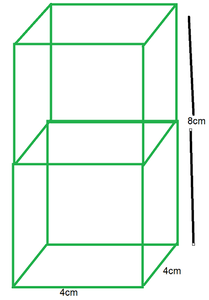### Question 2. A vessel is in the form of a hollow hemisphere mounted by a hollow cylinder. The diameter of the hemisphere is 14 cm and the total height of the vessel is 13 cm. Find the inner surface area of the vessel.

Solution:

Height of cylinder = 13-7 = 6 cm

Inner surface area of vessels=C.S.A of cylinder+ C.S.A of Hemisphere

=2πrh+2πr2

=2πr(h+r)

=2*22/7*7(6+7)

=44(13)

=572cm2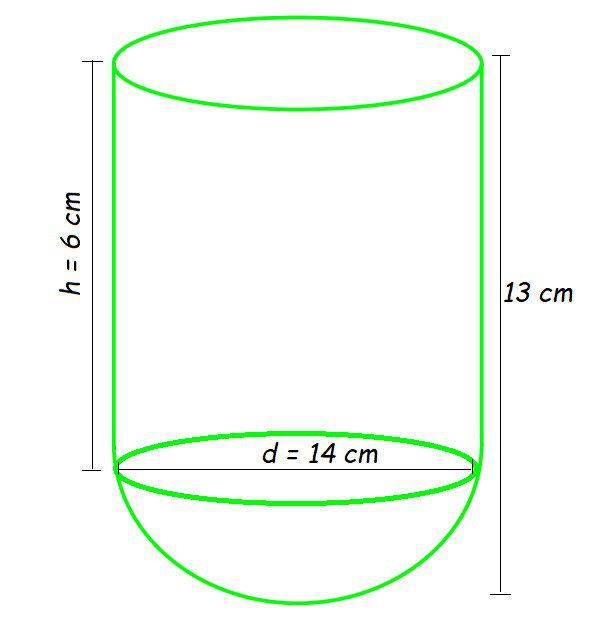### Question 3. A toy is in the form of a cone of radius 3.5 cm mounted on a hemisphere of same radius. The total height of the toy is 15.5 cm. Find the total surface area of the toy.

Solution:

Height of cone(h)=15.5-3=12cm

l=√(h2+r2)

l=√(122+3.52)

l=√(144+12.25)

=√256.25

=12.5cm

Total surface area of toy=C.S. A of cone+ C.S.A of hemisphere

=πrl+2πr2

=πr(l+2r)

=22/7*3.5(12.5+2(3.5))

=11(12.5*7)

=11(19.5)

=214.5cm2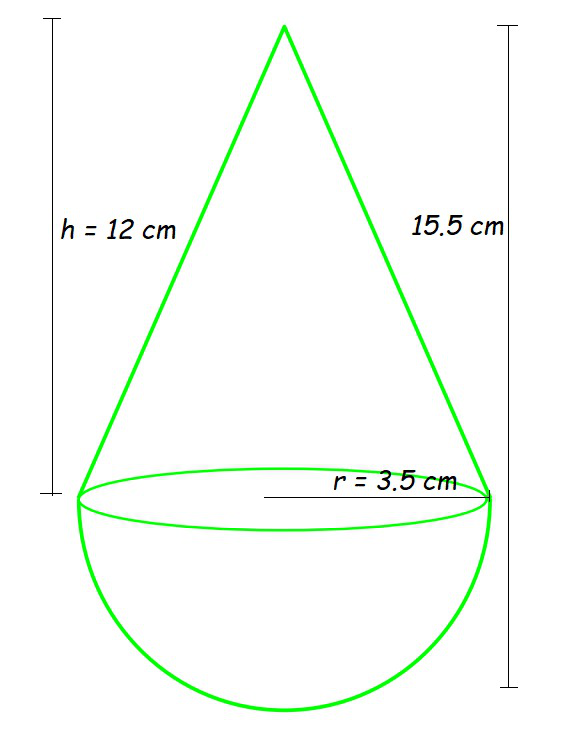### Question 4. A cubical block of side 7 cm is surmounted by a hemisphere. What is the greatest diameter the hemisphere can have? Find the surface area of the solid.

Solution:

Surface area of solid=T.S.A of cube-Area of circle+ C.S.A of hemisphere

=6*side*side-πr2+2πr2

=6*side*side+πr2

=6*7*7+22/7*7/2*7/2

=294+72/2

=294+38.5

=332.5cm2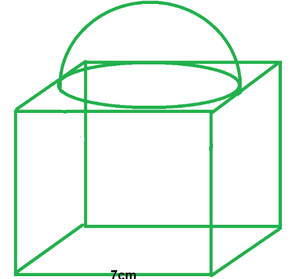### Question 5. A hemispherical depression is cut out from one face of a cubical wooden block such that the diameter l of the hemisphere is equal to the edge of the cube. Determine the surface area of the remaining solid.

Solution:

Surface area remaining solid=T.S.A of cube -Area of circle+ C.S.A of hemisphere

=6*side*side- πr2+2πr2

=6*l*l- πr2

=6l2-πr2

=6l2 – π(l/2)2

=6l2 – πl2/4

=(24l2+ πl2)/4

=l2(24+π)/4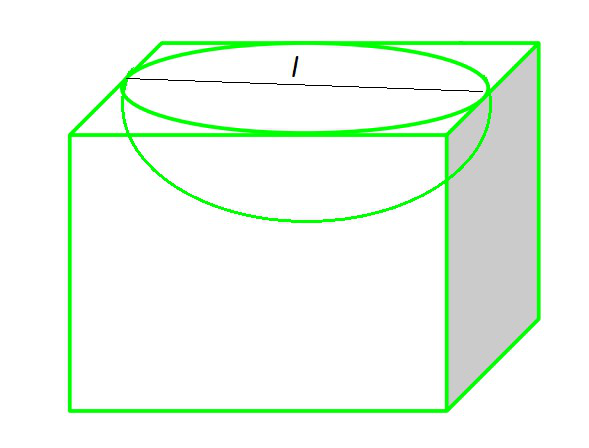### Question 6. A medicine capsule is in the shape of a cylinder with two hemispheres stuck to each of its ends. The length of the entire capsule is 14 mm and the diameter of the capsule is 5 mm. Find its surface area.

Solution:

D=5mm   r=5/2mm

h=14-5=9

Surface area of cylinder=C.S. A of cylinder+ C.S.A of 2 Hemisphere

=2πrh+2πr2*2

=2πr(h+2r)

=2*22/7*5/2(9+2*5/2)

=110/7(9+5)

=110/7*14

=220mm2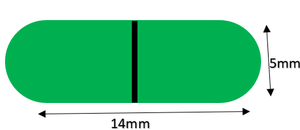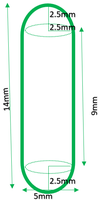### Question 7. A tent is in the shape of a cylinder surmounted by a conical top. If the height and diameter of the cylindrical part are 2.1 m and 4 m respectively, and the slant height of the top is 2.8 m, find the area of the canvas used for making the tent. Also, find the cost of the canvas of the tent at the rate of Rs 500 per m2. (Note that the base of the tent will not be covered with canvas.)

Solution:

i) d=4m r=4/2=2m

Area of canvas =C.S.A of cone+ C.S.A of cylinder

=πrl+2πrh

=πr(l+2h)

=22/7(2.8+2*2.1)

=44*(2.8+4.2)/7

=44*7/7

=44m2

ii) cost of canvas=Area*rate

=44m2*Rs. 500/m2

=Rs. 22,000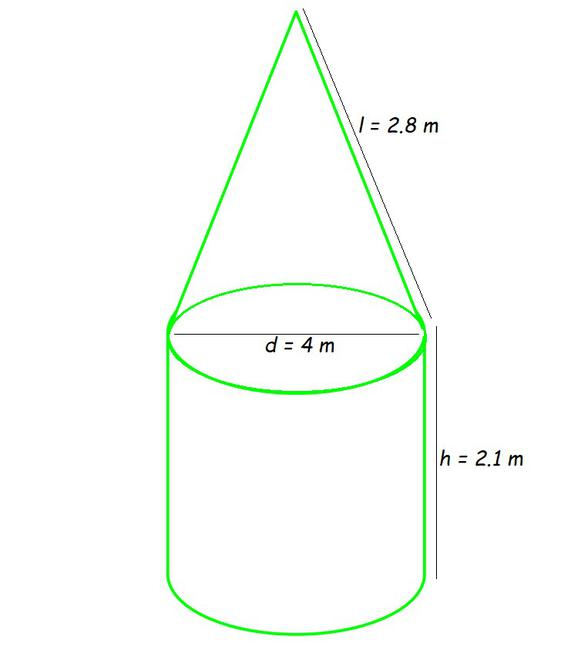### Question 8. From a solid cylinder whose height is 2.4 cm and diameter 1.4 cm, a conical cavity of the same height and same diameter is hollowed out. Find the total surface area of the remaining solid to the nearest cm2.

Solution:

D=1.4 cm,   r=1.4/2=14/20=7/10=0.7

l= √(h2+r2)

= √((2.4)2+(0.7)2)

= √(5.76+0.49)

= √6.25

= 2.5cm2

Total surface area of remaining solid=C.S.A of cylinder+ C.S.A of cone+ Area of circular base

= 2πrh+πrl+πr2

= πr(2h+l+r)

= 22/7*7/10(2(2.4)+2.5+0.7)

= 22(4.8+2.5+0.7)/10

= 22(8)/10

= 176/10

= 17.6cm2

Nearest ten=18cm2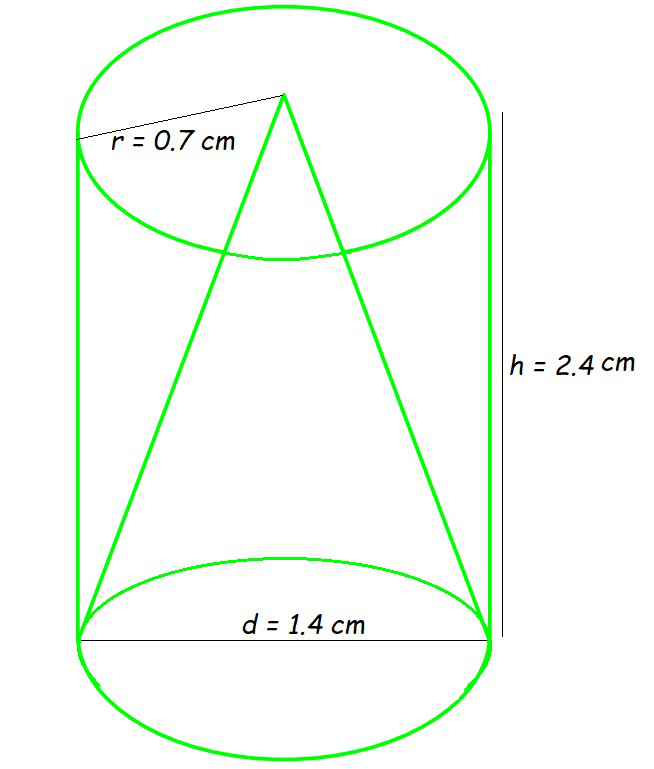### Question 9. A wooden article was made by scooping out a hemisphere from each end of a solid cylinder, as shown in figure. If the height of the cylinder is 10 cm and its base is of radius 3.5 cm, find the total surface area of the article.

Solution:

Total surface area of article=C.S.A of cylinder+2*C.S.A of hemisphere

=2πrh+2*2πr2

=2πr(h+2r)

=2*22/7*3.5(10+2(3.5))

=22(10+7)

=22(17)

=374cm2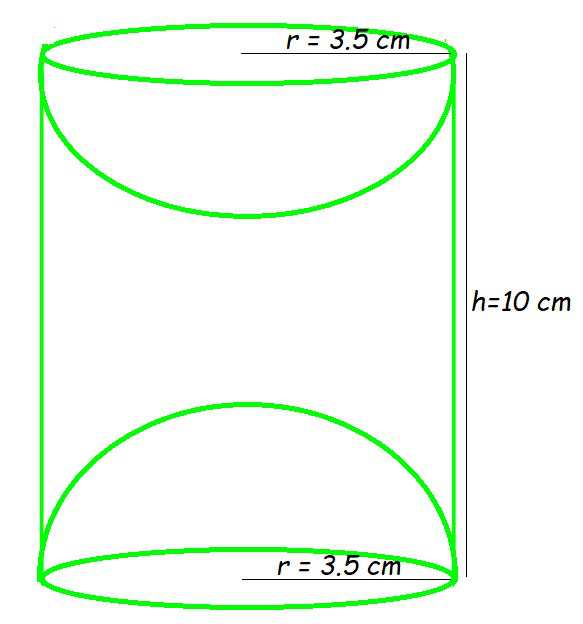My Personal Notes arrow_drop_up